International
Tables for
Crystallography
Volume C
Mathematical, physical and chemical tables
Edited by E. Prince

International Tables for Crystallography (2006). Vol. C, ch. 6.1, pp. 593-595

## Section 6.1.3. Nuclear scattering of neutrons

B. T. M. Willisd

### 6.1.3. Nuclear scattering of neutrons

| top | pdf |

#### 6.1.3.1. Glossary of symbols

| top | pdf |

 b Bound nuclear scattering length bfree Free nuclear scattering length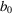Potential scattering length b′, b′′ Real and imaginary parts of resonant scattering length bcoh Coherent scattering length F(h) Structure factor for nuclear Bragg scattering 2πh Reciprocal-lattice vector H Scattering vector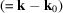I Nuclear spin k Wavevector of scattered neutronWavevector of incident neutron M Nuclear massNeutron mass N Number of unit cells in crystal V Volume of unit cell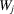Exponent of temperature factor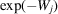of jth atom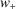Weight of spin state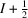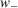Weight of spin state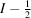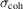Coherent scattering cross section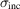Incoherent scattering cross section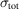Total scattering cross section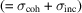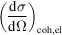Differential coherent elastic scattering cross section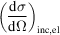Differential incoherent elastic scattering cross section

The nucleus is the fundamental unit involved in the scattering of neutrons by atoms. For magnetic materials, electronic scattering takes place as well (see Section 6.1.2). Apart from these two main interactions, there are a number of subsidiary ones (Shull, 1967) that are extremely weak and can be ignored in nearly all diffraction studies.

In this section, we discuss the neutron–nucleus interaction only, starting from scattering by a single nucleus, then scattering by an atom, and finally scattering by a single crystal. For a more detailed account, see Bacon (1975).

#### 6.1.3.2. Scattering by a single nucleus

| top | pdf |

The nuclear forces giving rise to the scattering of neutrons have a range of 10−14 to 10−15 m. This is much smaller than the wavelength of thermal neutrons, and so (from elementary diffraction theory) the neutron wave scattered by the nucleus is spherically symmetrical. Unlike magnetic scattering, there is no `form-factor' dependence of nuclear scattering on the scattering angle.

The incident neutron beam can be represented by the plane wave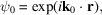withdenoting the wavevector of the neutron and r its position relative to the nucleus. Then, for a nucleus of zero spin, the wavefunction of the scattered neutron is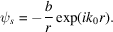b is the bound nuclear scattering length or nuclear scattering amplitude, and the negative sign ensures that b is positive for hard-sphere or potential scattering.

If the nucleus is free to recoil under the impact of the neutron, as in a gas, the scattering must be treated in the centre-of-mass system. The free scattering length is related to the bound scattering length b in condensed matter by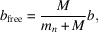where M is the nuclear mass andthe mass of the neutron. For hydrogen, 1H, the free scattering length is one half the bound scattering length, but the difference between the two rapidly diminishes for heavier nuclei.

In general, b is a complex quantity: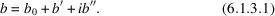is the scattering length associated with potential scattering, i.e. scattering in which the nucleus behaves like an impenetrable sphere. b′ and b′′ are the real and imaginary parts of the resonance scattering that takes place with the formation of a compound nucleus (nucleus plus neutron). Resonance scattering is only significant when the excitation energy of the neutron is close to an energy level of the compound nucleus. This occurs for relatively few nuclei, e.g. 113Cd, 149Sm, 157Gd, 176Lu, and b then varies rapidly with wavelength (Fig. 6.1.3.1). The phenomenon of resonance scattering has been used to phase neutron reflections (Schoenborn, 1975), but one difficulty is the strong absorption arising from the imaginary component b′′. For the majority of nuclei, the compound nucleus is not formed near resonance: the imaginary component is small, and the scattering length is independent of the neutron wavelength.Figure 6.1.3.1 | top | pdf |Dependence on neutron wavelength of the coherent scattering length of 113Cd. b0 is the potential scattering component, and b′ and b′′ the real and imaginary components of the resonance scattering. The resonance wavelength is 0.68 Å.

There is confusion in the literature regarding the appropriate signs for the real and imaginary parts of the scattering amplitude (Ramaseshan, Ramesh & Ranganath, 1975). The scattering-length curves in Fig. 6.1.3.1have been drawn to be consistent with the structure-factor formulae in Volume A (IT A, 2005).

Consider now the scattering from a nucleus with non-zero spin I. The neutron has spin, and the spin of the combined nucleus–neutron system is eitheror. Each spin state has its own scattering length, b+ or b, and the weights of these states (for scattering unpolarized neutrons) are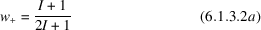and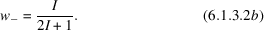Values of b+ and b have been determined experimentally for just a few nuclei with non-zero spin: 1H, 2H, 23Na, 59Co, ….

#### 6.1.3.3. Scattering by a single atom

| top | pdf |

For a single element containing several isotopes, each isotope has its own characteristic scattering length(s). The mean value of the scattering length of the atom is obtained by averaging (where necessary) over the two spin states of the isotope: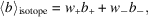where the angle brackets indicate a mean andandare given by (6.1.3.2);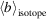is then averaged over all isotopes, taking into account their relative abundance. The resultant quantity,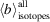, is known as the coherent scattering length of the atom, denoted bcoh. bcoh plays the same role in neutron scattering as the atomic scattering factor f in X-ray scattering. Table 4.4.4.1lists the coherent scattering lengths for the atoms in the Periodic Table.

The coherent scattering cross section of an atom is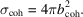It represents that part of the total scattering cross section, σtot, that gives interference effects with other atoms. The total cross section is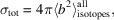and the incoherent scattering cross section, σinc, is the difference between σtot and σcoh: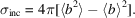In incoherent scattering, there is no phase relationship between the waves scattered by different atoms. σinc for hydrogen is 40 times larger than σcoh, but the proportion of coherent scattering is substantially increased by deuteration. The scattering from vanadium is almost entirely incoherent, and so it is useful as a container of polycrystalline samples.

#### 6.1.3.4. Scattering by a single crystal

| top | pdf |

The scattering from a single crystal can be either elastic or inelastic. An elastic process is one in which there is no exchange of energy between the neutron and the target nucleus. In an inelastic process, energy exchange occurs, giving rise to the creation or annihilation of elementary excitations such as phonons [see Section 4.1.1of Volume B (IT B, 2001)]. Here we shall be concerned only with elastic Bragg scattering.

If kinematic scattering conditions are assumed, the differential cross section,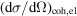, giving the probability of coherent elastic scattering by a single crystal into the solid angle, is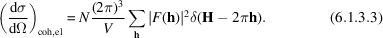Here, N is the number of unit cells, each of volume V, 2πh is a reciprocal-lattice vector, and F(h) is the nuclear structure factor for Bragg scattering. H is the scattering vector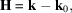where k and(with k = k0 = 2π/λ) are the wavevectors of the scattered and incident beams, respectively, and the δ function indicates that the coherent elastic scattering is simply Bragg scattering. F(h) is defined by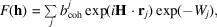in which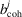is the coherent scattering length of the jth atom in the unit cell,is its equilibrium position with respect to the cell origin, and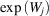its Debye–Waller temperature factor.

The incoherent elastic scattering cross section is given by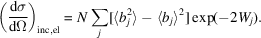Apart from the influence of the Debye–Waller temperature factor, this expression shows that the incoherent scattering is distributed uniformly throughout reciprocal space.

### References

Bacon, G. E. (1975). Neutron diffraction, 3rd ed. Oxford: Clarendon Press.
International Tables for Crystallography (2005). Vol. A, edited by Th. Hahn. Heidelberg: Springer.
International Tables for Crystallography (2001). Vol. B, edited by U. Shmueli, 2nd ed. Dordrecht: Kluwer Academic Publishers.
Ramaseshan, S., Ramesh, T. G. & Ranganath, G. S. (1975). A unified approach to the theory of anomalous scattering. Some novel applications of the multiple-wavelength method. Anomalous scattering, edited by S. Ramaseshan & S. C. Abrahams, pp. 139–161. Copenhagen: Munksgaard.
Schoenborn, B. P. (1975). Phasing of neutron protein data by anomalous dispersion. Anomalous scattering, edited by S. Ramaseshan & S. C. Abrahams, pp. 407–421. Copenhagen: Munksgaard.
Shull, C. G. (1967). Neutron interactions with atoms. Trans Am. Crystallogr. Assoc. 3, 1–16.